# Robot Localization and the Particle Filter

Most robots operate in environments that are rife with disturbances. Things like wind, dynamic environments, even faults in the robot hardware, can cause it to deviate from a desired path. As a result, in order for a robot to operate effectively, it needs to be able to determine its location with high accuracy and precision. This process is called localization, and it enables a robot to operate confidently in an environment which is constantly undermining the robot’s ability to perform its task.

Modern localization techniques in robotics and other autonomous systems utilize sensors on the robot that allow it to perceive its environment. A robot can then utilize this knowledge to make an informed estimate of its location/orientation, which it can then use to plan its next maneuver. A popular algorithm for localization called the Particle Filter, which is a concrete implementation of the more abstract Bayes Filter, allows a robot to

1. Efficiently represent its belief of where it is
2. Utilize sensor measurements to improve this belief

In this discussion, we will explore the intuition behind the Bayes Filter, discuss how the Particle Filter implements the Bayes Filter, and then demonstrate its value through a simulation of a 2D mobile robot.

Note: The Julia code for this simulation can be found here.

# Robot Terminology and Notation

Let us start this discussion with some definitions and terminologies.

The state of a robot is a mathematical object that allows us to perfectly describe the robot at a given point in time. Denoted as x, it is most often a vector whose elements are quantities that describe the position, orientation, etc. of the robot. A robot state is not unique, many different states can all be used to represent the same robot. Selection/definition of the form of the state is usually chosen to simplify certain aspects of the problem.

A measurement is another mathematical object that describes the information recorded by the sensors of the robot. It is also most often a vector containing all the different readings from the sensors, like speedometers, rangefinders, GPS receivers, etc. We will denote a single measurement vector at a point in time as z.

Almost all physical robots operate continuously in time, so the state of the robot is actually a continuous vector-valued function of time. However working with this kind of representation is computationally intractable, so we almost always discretize time into finite points. We will use the subscript t to denote states or measurements at a specific point in time t. The subscript t-1 refers to the previous point in time, relative to time t.

# Robot State as a Probability Distribution

As we discussed at the start, we are almost never exactly certain of the state of the robot at a point in time. This necessitates the representation of the robot state as a probability distribution over the state space, rather than just a single vector.

Note: The state space is the set of all possible states of the robot.

This allows us to represent what we think the robot state is, and it also allows us to represent our uncertainty in this belief as well. For this reason, I will use the word “belief” and “probability distribution” interchangeably in the context of the robot state.

# Bayes Filter

Bayes Rule, shown in the equation below, is the foundation behind many of the best localization algorithms (among a very many other things outside the field of robotics).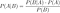Bayes Rule

It describes how you can update a probability distribution using an observation that is related to the quantity of interest that the distribution describes. This fits our needs for localization perfectly, as it allows us to update our belief of the robot’s state P(x) using sensor measurements z.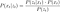Bayes rule for probabilistic robots

The algorithm for the Bayes Filter is composed of two steps. First, we use the state transition model (we will talk about this soon) to compute our belief of the current state using the belief of the previous state, but without considering the sensor measurements.Bayes Filter

This intermediate belief is denoted with a bar over the x. Then, we use Bayes Rule and the sensor measurement to update the belief and hopefully reduce the uncertainty by increasing the concentration of the probability mass towards the actual state of the robot.

That’s all there is to it. We repeat this process, continually taking sensor measurements and updating our belief of the robot state as it moves around in its environment. Can it really be this simple?

Well, not quite. The difficulty arises in how we choose to represent the belief of the state. One of the most popular implementations of the Bayes Filter is the Kalman Filter, where we represent the belief as a multivariate normal distribution. This is obviously a very big simplification, but it does provide very good results for many robots, especially in its more sophisticated forms like the Extended Kalman Filter.

The Particle Filter is another implementation that allows us to represent arbitrary beliefs with arbitrary accuracy. We will describe how it works and then test it out in simulation.

# Particle Filter

The Particle Filter represents a probability distribution using a set of “particles”, which can each be thought of as a sample from the distribution. For the belief of the state, every particle is a possible state, and the collection of the particles represents the belief. This allows us to represent the belief with arbitrary accuracy, by simply increasing the number of particles. In other words, the algorithms allows us to directly trade computational efficiency for accuracy.

Note: If we are interested in reconstructing the full belief from the particles, we could use a technique like Kernel Density Estimation. However, in practice we don’t ever need the full belief in order to determine the most likely location; oftentimes the mean or the geometric median of the particles suffices at a way to get a single most likely state from the belief.

Each iteration of the Particle Filter involves three steps:

1. Transform every particle using the state transition model
2. Compute a weight for each particle as the probability of observing the actual sensor measurement conditioned on the particle, p(z|x)
3. Sample from the particles with replacement, with probabilities proportional to the weights

Lets examine each step and see what it corresponds to in the Bayes Filter. The first step is to apply the state transition model to every particle in the set of particles. I’ve mentioned this “state transition model” a couple times now, so I will explain what it is.

The state transition model is a function from the state space to the state space. Its input is a state at time t-1, and the output is the state at time t. This function is a random function, so if we evaluate it multiple times with the same input, we will get different outputs. It is a model for how the robot moves at each point in time, and is random due to the disturbances present during robot movements.

Note: The state transition model may not accurately describe how the robot moves. It is a model, and therefore necessarily makes assumptions on the kinematics/dynamics of the robot as well as the sources of disturbances. The “randomness” involved in the function is how we have modeled the disturbances, and is not guaranteed to be accurate at all. It is usually developed by observing how the robot moves in the real world and observing how it is disturbed. These observations are then used to parameterize the disturbances when building the model.

This first step in the Particle Filter is therefore the implementation of the first step in the Bayes filter.

The second and third steps of the Particle Filter implement the second step in the Bayes Filter. To be specific, the resampling of the particles with probabilities proportional to their weights is the method by which we are incorporating the sensor measurements to improve the belief. This is because the weight of each particle is the probability of having observed the sensor measurement that we measured, if the true state were indeed the state of that particle. If this probability is low, then the weight will be low, which means that that particle represents an unlikely state. Its weight will therefore be low, which means that it will be unlikely to “survive” the resampling process. On the other hand, if the probability of having observed the sensor measurement is high, that means that the particle represents a likely state. The weight for this particle will therefore be high, which means that the particle is likely to end up in the new sample, possible more than once.

Through this sampling process, we are removing unlikely states and supporting likely states through possible duplication. The end result should hopefully be that the particles cluster more tightly around the actual state after the sampling process than before.

Note: This is actually very similar to a class of optimization algorithms called genetic algorithms, where possible solutions are considered as “organisms” that procreate to combine their properties and are subject to an adversarial process at each “generation” that removes the worst performing solutions.

# A 2D Mobile Robot

To test out the particle filter, we will simulate a simple 2D mobile robot. The robot moves along the ground in the direction that its facing, and can also turn to change that direction. The state transition model that we use to model the robot is the kinematic model for a 2D mobile robot described in the book “Probabilistic Robotics” by Wolfram Burgard, Dieter Fox, and Sebastian Thrun. The state of the robot is a three-element vector, whose first two entries describe its location, and whose last element describe the direction its facing.

In addition to the state transition model, we also need a model for the sensors of the robot, so we can compute p(z|x) in the particle filter. The sensors we use for the robot are an array of rangefinders, each one pointed in a direction around the robot. Each sensor is a time-of-flight sensor, which means that it transmits a beam of light (usually outside the visible spectrum) along the direction of measurement, and then counts how long it takes for the beam to be reflected off an object and returned to the sensor. Using the speed of light and this recorded time, the sensor can compute the distance to the target. This type of sensor is not without noise however, so we need a way to model the expected noise. We assume that one of four things happens when the sensor makes a measurement:

1. The beam hits the object and returns properly to the sensor. There is some noise in the measurement modeled by a normal distribution.
2. The beam fails to return to the sensor, in which case the sensor returns a maximum range measurement. We model this with a point mass distribution.
3. The beam is returned early to the sensor before it hits the physical obstacle, due to an interaction with something in the atmosphere. We model this with an exponential distribution.
4. The sensor has some other problem, in which case it returns a range that is uniformly distributed from zero to the maximum range.

All four of these possibilities (let’s call them modes) can each happen with some nonzero probability, and if we combine the probability distributions of each mode using an appropriate set of weights that describe the likelihood of each mode, then we can obtain a single distribution for the sensor measurement. Shown below is an instance of this distribution along with a histogram of samples drawn from the distribution.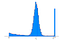PDF of sensor measurements and histogram of sensor samples

We see that the majority of sensor measurements are near the actual range, denoted with the asterisk, and a the rest of the measurements occur from one of the other three failure modes. This sensor model is also described in more detail in the book “Probabilistic Robotics”.

Now that we have our state transition model and sensor model, we are ready to simulate the robot.

# Simulation

We place the robot in its initial configuration and we initialize 1000 particles using the initial state. We initially make all the particles the same to represent a perfect confidence in the robot’s initial location.

Note: In the real world, this isn’t usually the case; there might be some uncertainty in the robot’s initial location (we might not even know it at all, in which case the particles will be uniformly distributed over the entire state space).

In addition, we also place a box near the robot within the range of one of its sensors. We “program” the robot to make a left turn around the box. In this simulation and the following ones, we won’t control the robot intelligently. In other words the robot just blindly follows a turn order. Obviously in the real world, the control programmed in the robot would be in the form of a goal or a path for the robot, but for simplicity, our robot will just “blindly” follow a command. Shown below is the result of the simulation for five steps, without making sensor measurements.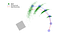Simulation of the robot with no sensor updates

The red line in the figure is the “dead reckoning” line, which is the line that the robot would have followed if it wasn’t being disturbed when it moved. It also represents the most naive belief in the actual path of the robot, if we never made any of the probabilistic considerations we have been discussing. The blue line is the “ground truth”, or the actual path that the robot took, and we can see that it deviates from the “dead reckoning” path due to the simulated disturbances. The green dots represent the particles at each step of the simulation

Note: The green dots are actually the projection of the particles onto the first two coordinates of the state space, and they don’t convey any information about the belief of the orientation of the robot. Rest assured that the actual particles include this information, we just omit it for the purpose of visualizing the collection of particles.

From the increasing spread of the particles, we can see that the robot is steadily becoming more uncertain about its location. If we allowed this to continue for more steps, the robot would eventually lose all certainty in its location as the points begin to rapidly spread out over the state space. To prevent this, we will turn on the sensors, and use the Particle Filter to update the belief at each step.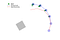Simulation of the robot with sensor updates

Using the sensor measurements, the robot is able to prevent the rise in uncertainty at each step. The particles are still spreading, but at a much slower rate. Even after five steps, the uncertainty in the location is still fairly low. The reason that it keeps increasing, even with the sensor updates, is that the sensors aren’t perfect. The noise present in the sensors keeps the particle filter from reducing the uncertainty arbitrarily low, i.e. the noise of the sensor places a bound on our ability to reduce the uncertainty.

Lets add more obstacles to the environment and increase the simulation time. We also program the robot to follow the initial left turn with a similar right turn.

We see that the robot has severely deviated from the “dead reckoning” path due to the accumulation of the disturbances. However, even though the robot has “fallen off the path”, it knows that it has. This is the crucial detail that exposes the importance of the Bayes Filter. Before we can even begin to think about how the robot can get back on the desired path, it needs to know where it is. The filtering process and sensor updates allow the robot to keep track of where it is, which can enable it to correct for the disturbances.

# Conclusion

We’ve explored the Bayes Filter and the Particle Filter, and hopefully demonstrated its usefulness for robot localization. There’s a whole lot more to the field of robot localization, but the end goal is always the same: to determine with the highest degree of confidence, where the robot is. The Bayes Filter and its many derivative like the Particle Filter have enabled us, and will continue to enable us, to build robots to do amazing things.

M.S. Student in Data Science @ Brown University, interested in Robotics, Aerospace Engineering, and anything simulated.

## More from Maitreya Venkataswamy

M.S. Student in Data Science @ Brown University, interested in Robotics, Aerospace Engineering, and anything simulated.